##### Accounting: 1,001 Practice Problems For DummiesGross margin and contribution margin tell two different stories based on the same sales numbers. To calculate the gross margin, you subtract cost of goods sold from sales. To find the contribution margin, you subtract cost of goods sold and variable costs from sales.

The following practice problems show the results for the two types of margins.

## Practice questions

Answer the following questions using the below information.

Exotic Case has the following information available in its internal reporting system:

• Sales volume, in units: 47,350

• Selling price per unit: \$300

• Cost of goods sold per unit: \$140

• Variable costs associated with each unit (sales commission): \$30

• Fixed costs: \$3,500,000

1. Calculate the gross profit, also called the gross margin.

2. Calculate the contribution margin.

1. \$7,576,000

The difference between sales and cost of goods sold is gross profit, sometimes called gross margin. Sales equal the number of units sold times the selling price.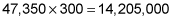Cost of goods sold equals the number of units sold times the cost per unit.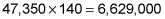Sales minus cost of goods sold: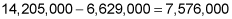2. \$6,155,500

Sales revenue less all variable costs, which includes cost of goods sold and other variable costs, equals the contribution margin. Sales revenue equals the number of units sold times the selling price.Cost of goods sold equals the number of units sold times the cost per unit.Total variable costs equal the number of units sold times the variable cost per unit.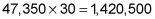Sales less cost of goods sold less total variable costs equals the contribution margin.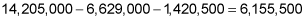If you need more practice on this and other topics from your accounting course, visit Dummies.com to purchase Accounting For Dummies! Featuring the latest information on accounting methods and standards, the information in Accounting For Dummies is valuable for anyone studying or working in the fields of accounting or finance.